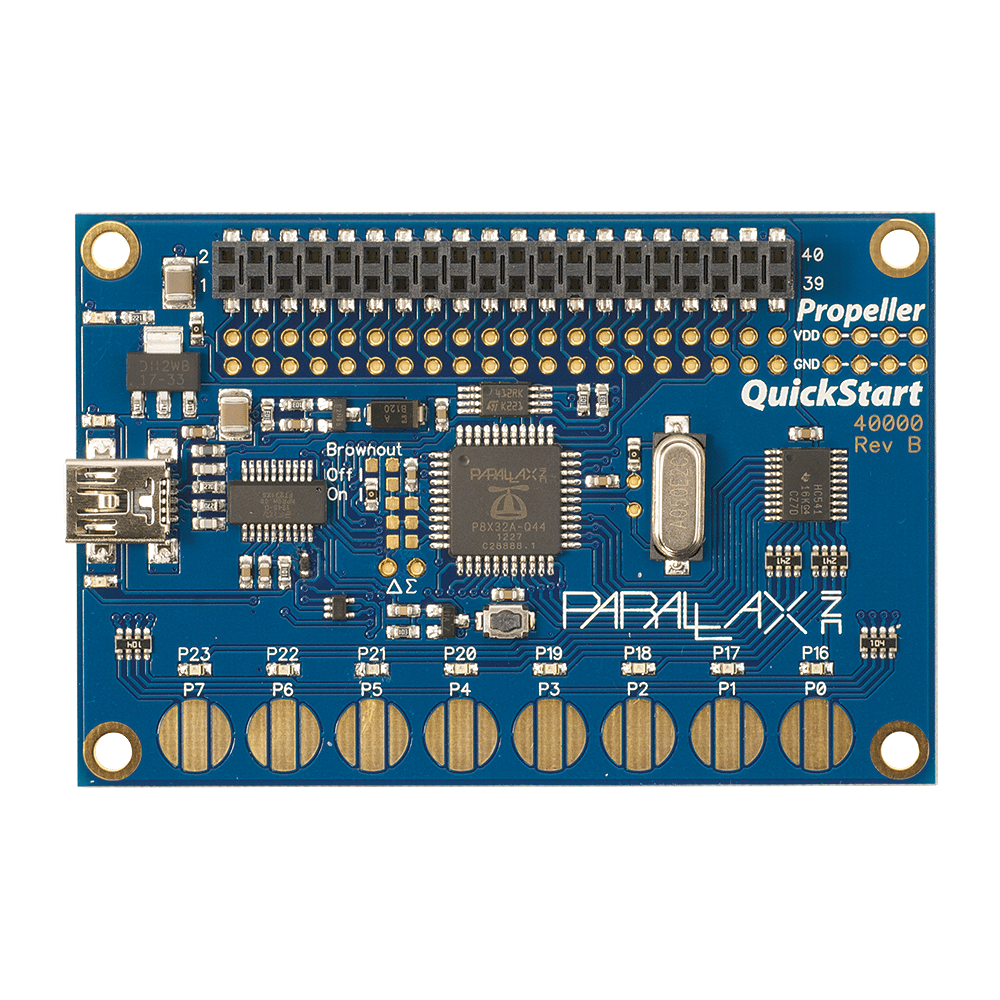PWM Measurement — Parallax Forums

# PWM Measurement

edited 2021-04-08 13:21

Hello my friends,

I have a problem with the PWM measurement. I cannot on my parallax card.

scenario:

We have 3 different PWM inputs and we want to measure them separately. How can I write this code? We need to see how many milliseconds are 1 and how many milliseconds are 0.## Comments

• Here's a very simplistic approach:

```pub measure_cycle(pin, p_high, p_low) | mask, t0, t1, t2

mask := 1 << pin

dira[pin] := 0

if (ina[pin] == 0)
waitpeq(mask, mask, 0)
t0 := cnt
waitpne(mask, mask, 0)
t1 := cnt
waitpeq(mask, mask, 0)
t2 := cnt
long[p_high] := (t1 - t0) / MS_001
long[p_low]  := (t2 - t1) / MS_001

else
waitpne(mask, mask, 0)
t0 := cnt
waitpeq(mask, mask, 0)
t1 := cnt
waitpne(mask, mask, 0)
t2 := cnt
long[p_low]  := (t1 - t0) / MS_001
long[p_high] := (t2 - t1) / MS_001
```

You need to pass pointers to your high and low milliseconds variables, and define the MS_001 constant as the number of system ticks in one millisecond. Probably better, though, to use the system counters to measure the low and high periods. I wrote the attached object to measure the duty cycle output from a DMX lighting controller. This was used in a costume for a television show. The lighting operator changed the levels out of the DMX receiver which were translated by the Propeller into lighting and animation commands for the costume piece (Thunder's buckle from the TV show "Black Lightning"). I use the counters to measure the high and low periods of the cycle.

Sign In or Register to comment.